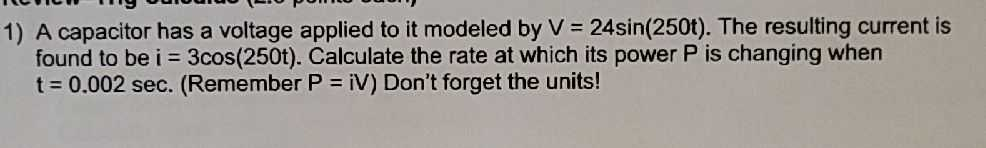### ¿Todavía tienes preguntas de matemáticas?

Pregunte a nuestros tutores expertos
Algebra
PreguntaA capacitor has a voltage applied to it modeled by $$V = 24 \sin ( 250 t )$$ . The resulting current is found to be $$i = 3 \cos ( 250 t )$$ . Calculate the rate at which its power $$P$$ is changing when

$$t = 0.002 sec$$ . (Remember $$P = iV$$ ) Don't forget the units!